Ten fractions

Write ten fractions between 1/3 and 2/3

Result

a1 =  0.367
a2 =  0.383
a3 =  0.4
a4 =  0.417
a5 =  0.433
a6 =  0.45
a7 =  0.467
a8 =  0.483
a9 =  0.5
a10 =  0.517

Solution:Leave us a comment of example and its solution (i.e. if it is still somewhat unclear...):Be the first to comment!To solve this example are needed these knowledge from mathematics:

Need help calculate sum, simplify or multiply fractions? Try our fraction calculator.

Next similar examples:

1. Pizza 5You have 2/4 of a pizza and you want to share it equally between 2 people how much pizza does each person get?
2. Cake 71/3 of a cake shared with 4 people. What share of the whole cake has each people?
3. Women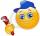In an company of 1050 employees are 2/3 women. How many women work in the company?
4. ChocolateChildren break chocolate first to third and then every part of another half. What kind got each child? Draw a picture. What part would have received if each piece have halved?
5. Balls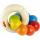The boys changed stamps, beads and balls. For 9 balls is 3 stamps, 2 balls is 44 stamps. How many beads is for 1 ball?
6. Product of two fractionsProduct of two fractions is 9 3/5 . If one of the fraction is 9 3/7. Find the other fraction.
7. Fractions 4How many 2/3s are in 6?
8. Icecreams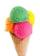Karthik bought 30 icecreams for his birthday .2/5 of them are chocolate icecreams. Find the number of chocolate icecreams he bought?
9. Rolls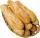Mom bought 13 rolls. Dad ate 3.5 rolls. How many rolls left when Peter yet put two at dinner?
10. CakesOn the bowl were a few cakes. Jane ate one-third of them, Dana ate a quarter of those cakes that remained. a) What part (of the original number of cakes) Dana ate? b) At least how many cakes could be (initially) on thebowl?
11. DoctorsIn the city operates 171 doctors. The city has 128934 citizens. How many citizens are per one doctor?
12. Dividing by five and tenNumber 5040 divide by the number 5 and by number 10: a = 5040: 5 b = 5040: 10
13. The resultHow many times I decrease the number 1632 to get the result 24?
14. On the farm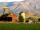The farm took 221 tons of potatoes from 17 hectares. How many tons of potatoes taken from one hectare?
15. Math classificationIn 3A class are 27 students. One-third got a B in math and the rest got A. How many students received a B in math?
16. Compare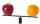Compare with characters >, <, =: 85.57 ? 80.83
17. Angles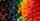Which of those angles are obtuse?